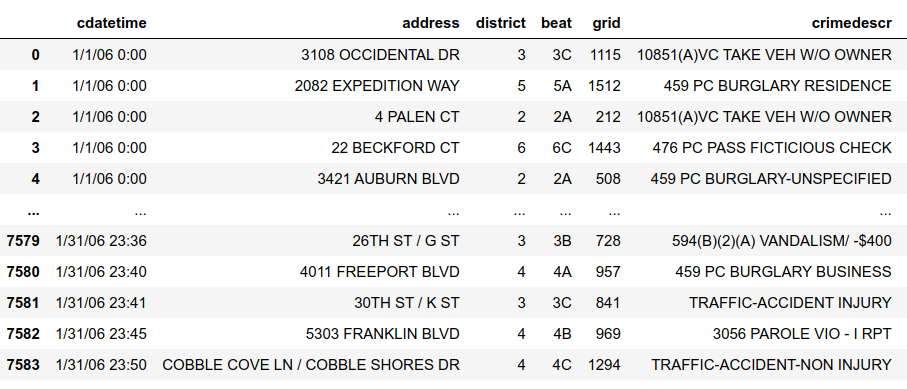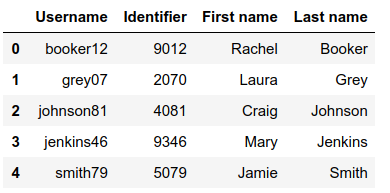Open in App
Not now

# Read multiple CSV files into separate DataFrames in Python

• Last Updated : 05 May, 2022

In this article, we will see how to read multiple CSV files into separate DataFrames. For reading only one data frame we can use pd.read_csv() function of pandas. It takes a path as input and returns data frame like

`df = pd.read_csv("file path")`

Let’s have a look at how it works

## Python3

 `# import module``import` `pandas as pd` `# read dataset``df ``=` `pd.read_csv(``"./csv/crime.csv"``)`

Here, crime.csv is the file in the current folder. CSV is the folder that contains the crime.csv file and CSV Reader.ipynb is the file containing the above code.

Output:It is the data frame that is read from the above function. One more file is present in the folder named – username.csv. To read them both and store them in different data frames use the below code

## Python3

 `# import module``import` `pandas as pd` `# assign dataset names``list_of_names ``=` `[``'crime'``,``'username'``]` `# create empty list``dataframes_list ``=` `[]` `# append datasets into the list``for` `i ``in` `range``(``len``(list_of_names)):``    ``temp_df ``=` `pd.read_csv(``"./csv/"``+``list_of_names[i]``+``".csv"``)``    ``dataframes_list.append(temp_df)`

dataframes_list contains all the data frames separately.

dataframes_list:dataframes_list:Here is another approach, now suppose that there are many files, and we don’t know the names and number then, use the below code

## Python3

 `# import modules``import` `os``import` `pandas as pd` `# assign path``path, dirs, files ``=` `next``(os.walk(``"./csv/"``))``file_count ``=` `len``(files)``# create empty list``dataframes_list ``=` `[]` `# append datasets to the list``for` `i ``in` `range``(file_count):``    ``temp_df ``=` `pd.read_csv(``"./csv/"``+``files[i])``    ``dataframes_list.append(temp_df)``    ` `# display datasets``for` `dataset ``in` `dataframes_list:``    ``display(dataset)`

Output:My Personal Notes arrow_drop_up